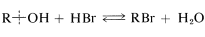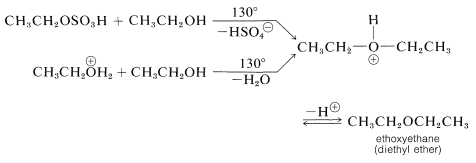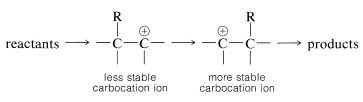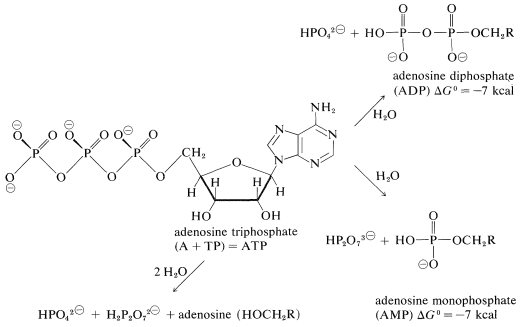# 15.6: Reactions Involving the C-O Bond of Alcohols

$$\newcommand{\vecs}{\overset { \rightharpoonup} {\mathbf{#1}} }$$ $$\newcommand{\vecd}{\overset{-\!-\!\rightharpoonup}{\vphantom{a}\smash {#1}}}$$$$\newcommand{\id}{\mathrm{id}}$$ $$\newcommand{\Span}{\mathrm{span}}$$ $$\newcommand{\kernel}{\mathrm{null}\,}$$ $$\newcommand{\range}{\mathrm{range}\,}$$ $$\newcommand{\RealPart}{\mathrm{Re}}$$ $$\newcommand{\ImaginaryPart}{\mathrm{Im}}$$ $$\newcommand{\Argument}{\mathrm{Arg}}$$ $$\newcommand{\norm}{\| #1 \|}$$ $$\newcommand{\inner}{\langle #1, #2 \rangle}$$ $$\newcommand{\Span}{\mathrm{span}}$$ $$\newcommand{\id}{\mathrm{id}}$$ $$\newcommand{\Span}{\mathrm{span}}$$ $$\newcommand{\kernel}{\mathrm{null}\,}$$ $$\newcommand{\range}{\mathrm{range}\,}$$ $$\newcommand{\RealPart}{\mathrm{Re}}$$ $$\newcommand{\ImaginaryPart}{\mathrm{Im}}$$ $$\newcommand{\Argument}{\mathrm{Arg}}$$ $$\newcommand{\norm}{\| #1 \|}$$ $$\newcommand{\inner}{\langle #1, #2 \rangle}$$ $$\newcommand{\Span}{\mathrm{span}}$$$$\newcommand{\AA}{\unicode[.8,0]{x212B}}$$

## Electrophilic Properties of Alcohols

Alkyl halide formation from an alcohol and a hydrogen halide affords an important example of a reaction wherein the $$\ce{C-O}$$ bond of the alcohol is broken:The reaction is reversible and the favored direction depends on the water concentration. Primary bromides often are prepared best by passing dry hydrogen bromide into the alcohol heated to just slightly below its boiling point.

Halide formation proceeds at a useful rate only in the presence of strong acid, which can be furnished by excess hydrogen bromide or, usually and more economically, by sulfuric acid. The alcohol accepts a proton from the acid to give an alkyloxonium ion, which is more reactive in subsequent displacement with bromide ion than the alcohol (by either $$S_\text{N}2$$ or $$S_\text{N}1$$ mechanisms) because $$\ce{H_2O}$$ is a better leaving group than $$\ce{OH}^\ominus$$:orWhether the displacement reaction is an $$S_\text{N}1$$ or $$S_\text{N}2$$ process depends on the structure of the alcohol. In general, the primary alcohols are considered to react by $$S_\text{N}2$$ and the secondary and tertiary alcohols by $$S_\text{N}1$$ mechanisms.

Hydrogen chloride is less reactive than hydrogen bromide toward primary alcohols, and a catalyst (zinc chloride) may be required. A solution of zinc chloride in concentrated hydrochloric acid (Lucas reagent) is a convenient reagent to differentiate between primary, secondary, and tertiary alcohols with less than eight or so carbons. Tertiary alcohols react very rapidly to give an insoluble layer of alkyl chloride at room temperature. Secondary alcohols react in several minutes, whereas primary alcohols form chlorides only on heating. The order of reactivity is typical of $$S_\text{N}1$$ reactions. Zinc chloride probably assists in the breaking of the $$\ce{C-O}$$ bond of the alcohol much as silver ion aids ionization of $$\ce{RCl}$$ (Section 8-7D):Thionyl chloride, $$\ce{O=SCl_2}$$, is useful for the preparation of alkyl chlorides, especially when the use of strongly acidic reagents, such as zinc chloride and hydrochloric acid, is undesirable. Thionyl chloride can be regarded as the acid chloride of sulfurous acid, $$\ce{O=S(OH)_2}$$, and like most acid chlorides the halogen is displaced readily by alcohols. Addition of 1 mole of an alcohol to 1 mole of thionyl chloride gives an unstable alkyl chlorosulfite, which generally decomposes on mild heating to yield the alkyl chloride and sulfur dioxide:Chlorides can be prepared in this way from primary and secondary, but not tertiary, alcohols. In practice, an equivalent of a weak base, such as pyridine (azabenzene), is added to neutralize the hydrogen chloride that is formed. If the acid is not removed, undesirable degradation, elimination, and rearrangement reactions may occur.

The thionyl chloride reaction apparently can proceed from the alkyl chlorosulfite stage by more than one mechanism: an ionic $$S_\text{N}2$$ chain reaction with chloride ion,or an $$S_\text{N}1$$-like ionization and collapse of the resulting $$\ce{R}^\oplus \ce{Cl}^\ominus$$ ion pair to give $$\ce{RCl}$$:orObviously, the greater the $$S_\text{N}2$$ reactivity associated withthe better the $$S_\text{N}2$$ reaction will go and, conversely, if $$\ce{R}^\oplus$$ is formed easily fromthe $$S_\text{N}1$$ reaction is likely to be favored.

Other halides that are useful in converting alcohols to alkyl halides are $$\ce{PCl_5}$$, $$\ce{PCl_3}$$, $$\ce{PBr_3}$$, and $$\ce{PI_3}$$, which are acid halides of phosphorus oxyacids. As with thionyl chloride, a weak base often is used to facilitate the reaction. The base acts to neutralize the acid formed, and also to generate bromide ion for $$S_\text{N}$$ reactions:## Sulfate and Sulfonate Esters

It is possible to prefer esters of sulfuric acid by the reaction of an alcohol with the acid:The reaction is closely related to alkyl halide formation under strongly acidic conditions, whereby conversion of the alcohol to an oxonium salt is a first step:Conversion of the oxonium hydrogen sulfate to the ester probably proceeds by an $$S_\text{N}2$$ mechanism with primary alcohols and an $$S_\text{N}1$$ mechanism with tertiary alcohols:An alternative mechanism, which operates either in $$100\%$$, or in fuming sulfuric acid (which contains dissolved $$\ce{SO_3}$$), is addition of sulfur trioxide to the $$\ce{OH}$$ group:The sodium salts of alkyl hydrogen sulfate esters have useful properties as detergents if the alkyl group is large, $$\ce{C_{12}}$$ or so:The mechanism of detergent action will be considered in more detail in Chapter 18.

In principle, dialkyl sulfates could be formed by an $$S_\text{N}2$$ reaction between an alkyloxonium salt and an alkyl sulfate ion:Indeed, if methanol is heated with fuming sulfuric acid, dimethyl sulfate, $$\ce{CH_3O(SO_2)OCH_3}$$, is obtained; but other alcohols are better converted to dialkyl sulfates by oxidation of the corresponding dialkyl sulfites formed by the reaction of 1 mole of thionyl chloride $$\left( \ce{SOCl_2} \right)$$ with 2 moles of the alcohol:The reason that dialkyl sulfates seldom are prepared by direct reaction of the alcohol with $$\ce{H_2SO_4}$$ is that the mono esters react rapidly on heating to eliminate sulfuric acid and form alkenes, as explained in Section 15-5C.

Sulfonic acids, $$\ce{R-SO_2-OH}$$ or $$\ce{Ar-SO_2-OH}$$, are oxyacids of sulfur that resemble sulfuric acid, $$\ce{HO-SO_2-OH}$$, but in which sulfur is in a lower oxidation state.

Sulfonate esters are useful intermediates in displacement reactions (Section 8-7C) and provide a route for the conversion of an alcohol, $$\ce{ROH}$$, to $$\ce{RX}$$ by the sequence:Sulfonate esters usually are prepared through treatment of the alcohol with the acid chloride (sulfonyl chloride) in the presence of pyridine (azabenzene):## Dehydration of Alcohols with Strong Acids

In the reaction of an alcohol with hot concentrated sulfuric acid, the alcohol is dehydrated to an alkene:This is the reverse of acid-catalyzed hydration of alkenes discussed previously (Section 10-3E) and goes to completion if the alkene is allowed to distill out of the reaction mixture as it is formed. One mechanism of dehydration involves proton transfer from sulfuric acid to the alcohol, followed by an $$E2$$ reaction of hydrogen sulfate ion or water with the oxonium salt of the alcohol:Alternatively, the alkyl hydrogen sulfate could be formed and eliminate sulfuric acid by an $$E2$$ reaction:At lower temperatures the oxonium salt or the alkyl hydrogen sulfate may react by an $$S_\text{N}$$ displacement mechanism with excess alcohol in the reaction mixture, thereby forming a dialkyl ether. Although each step in the reaction is reversible, ether formation can be enhanced by distilling away the ether as fast as it forms. Diethyl ether is made commercially by this process:Most alcohols also will dehydrate at fairly high temperatures in the presence of solid catalysts such as silica gel or aluminum oxide to give alkenes or ethers. The behavior of ethanol is reasonably typical of primary alcohols and is summarized in the following equations:## $$\ce{C-O}$$ Bond Cleavage of Tertiary Alcohols

Tertiary alcohols react with sulfuric acid at much lower temperatures than do most primary or secondary alcohols. The reactions typically are $$S_\text{N}1$$ and $$E1$$ by way of a tertiary carbocation, as shown here for tert-butyl alcohol and sulfuric acid:2-Methylpropene can be removed from the reaction mixture by distillation and easily is made the principal product by appropriate adjustment of the reaction conditions. If the 2-methylpropene is not removed as it is formed, polymer and oxidation products become important. Sulfuric acid often is an unduly strenuous reagent for dehydration of tertiary alcohols. Potassium hydrogen sulfate, copper sulfate, iodine, phosphoric acid, or phosphorus pentoxide may give better results by causing less polymerization and less oxidative degradation which, with sulfuric acid, results in the formation of sulfur dioxide.

The $$S_\text{N}1$$-$$E1$$ behavior of tertiary alcohols in strong acids can be used to advantage in the preparation of tert-butyl ethers. If, for example, a mixture of tert-butyl alcohol and methanol is heated in the presence of sulfuric acid, the tertiary alcohol reacts rapidly but reversibly to produce 2-methylpropene by way of the tert-butyl cation. This cation can be trapped by the methanol to form tert-butyl methyl ether. High yields of ethers can be obtained in this way:## Carbocation Rearrangements

Rearrangement of the alkyl groups of alcohols is very common in dehydration, particularly in the presence of strong acids, which are conducive to carbocation formation. Typical examples showing both methyl and hydrogen migration follow:The key step in each such rearrangement is isomerization of a carbocation, as discussed in Section 8-9B. Under kinetic control, the final products always correspond to rearrangement of a less stable carbocation to a more stable carbocation. (Thermodynamic control may lead to quite different results, Section 10-4A.)In the dehydration of 3,3-dimethyl-2-butanol, a secondary carbocation is formed initially, which rearranges to a tertiary carbocation when a neighboring methyl group with its bonding electron pair migrates to the positive carbon. The charge is thereby transferred to the tertiary carbon:## Phosphate Esters

Phosphoric acid $$\left( \ce{H_3PO_4} \right)$$ often is used in place of sulfuric acid to dehydrate alcohols. This is because phosphoric acid is less destructive; it is both a weaker acid and a less powerful oxidizing agent than sulfuric acid. Dehydration probably proceeds by mechanisms similar to those described for sulfuric acid (Section 15-5C) and very likely through intermediate formation of a phosphate ester:The ester can eliminate $$\ce{H_3PO_4}$$, as sulfate esters eliminate $$\ce{H_2SO_4}$$, to give alkenes:The chemistry of phosphate esters is more complicated than that of sulfate esters because it is possible to have one, two, or three alkyl groups substituted for the acidic hydrogens of phosphoric acid:Also, phosphoric acid forms an extensive series of anhydrides (with $$\ce{P-O-P}$$ bonds), which further diversify the number and kind of phosphate esters. The most important phosphate esters are derivatives of mono-, di-, and triphosphoric acid (sometimes classified as ortho-, pyro-, and meta-phosphoric acids, respectively):The equilibrium between the esters of any of these phosphoric acids and water favors hydrolysis:However, phosphate esters are slow to hydrolyze in water (unless a catalyst is present). The difference in kinetic and thermodynamic stability of phosphate esters toward hydrolysis is used to great effect in biological systems.

Of particular importance is the conversion of much of the energy that results from photosynthesis, or from the oxidation of fats, carbohydrates, and proteins in cells into formation of phosphate ester bonds $$\left( \ce{C-O-P} \right)$$ or phosphate anhydride bonds $$\left( \ce{P-O-P} \right)$$. The energy so stored is used in other reactions, the net result of which is hydrolysis:The substance that is the immediate source of energy for many biological reactions is adenosine triphosphate (ATP). Although this is a rather large and complex molecule, the business end for the purpose of this discussion is the triphosphate group. Hydrolysis of this group can occur to give adenosine diphosphate (ADP), adenosine monophosphate (AMP), or adenosine itself:(The phosphate groups are repesented here as the major ionized form present at pH $$\cong 7$$ in solutions of ATP.)

All of these hydrolysis reactions are energetically favorable $$\left( \Delta G^0 < 0 \right)$$, but they do not occur directly because ATP reacts slowly with water. However, hydrolysis of ATP is the indirect result of other reactions in which it participates. For example, as we showed in Section 15-4D, equilibrium for the direct formation of an ester from a carboxylic acid and an alcohol in the liquid phase is not very favorable (Equation 15-2). However, if esterification can be coupled with ATP hydrolysis (Equation 15-3), the overall reaction (Equation 15-4) becomes much more favorable thermodynamically than is direct esterification.The ATP hydrolysis could be coupled to esterification (or other reactions) in a number of ways. The simplest would be to have the ATP convert one of the participants to a more reactive intermediate. For esterification, the reactive intermediate is an acyl derivative of AMP formed by the displacement of diphosphate from ATP:The acyl AMP is like an acyl chloride, $$\ce{RCOCl}$$, in having a leaving group (AMP) that can be displaced with an alcohol:The net result of the sequence in Equations 15-5 and 15-6 is esterification in accord with Equation 15-4. It is not a catalyzed esterification because in the process one molecule of ATP is converted to AMP and diphosphate for each molecule of ester formed. The AMP has to be reconverted to ATP to participate again. These reactions are carried on by cells under the catalytic influence of enzymes. The adenosine part of the molecule is critical for the specificity of action by these enzymes. Just how these enzymes function obviously is of great interest and importance.

If the role of phosphate esters, such as ATP, in carrying out reactions such as esterification in aqueous media under the influence of enzymes in cells is not clear to you, think about how you would try to carry out an esterification of ethanol in dilute water solution. Remember that, with water in great excess, the equilibrium will be quite unfavorable for the esterification reaction of Equation 15-2. You might consider adding $$\ce{CH_3COCl}$$, for which the equilibrium for ester formation is much more favorable (Section 15-4D). However, $$\ce{CH_3COCl}$$ reacts violently with water to form $$\ce{CH_3CO_2H}$$, and this reaction destroys the $$\ce{CH_3COCl}$$ before it has much chance to react with ethanol to give the ester. Clearly, what you would need is a reagent that will convert $$\ce{CH_3CO_2H}$$ into something that will react with ethanol in water to give the ester with a favorable equilibrium constant and yet not react very fast with water. The phosphate esters provide this function in biochemical systems by being quite unreactive to water but able to react with carboxylic acids under the influence of enzymes to give acyl phosphates. These acyl phosphates then can react with alcohols under the influence of other enzymes to form esters in the presence of water.